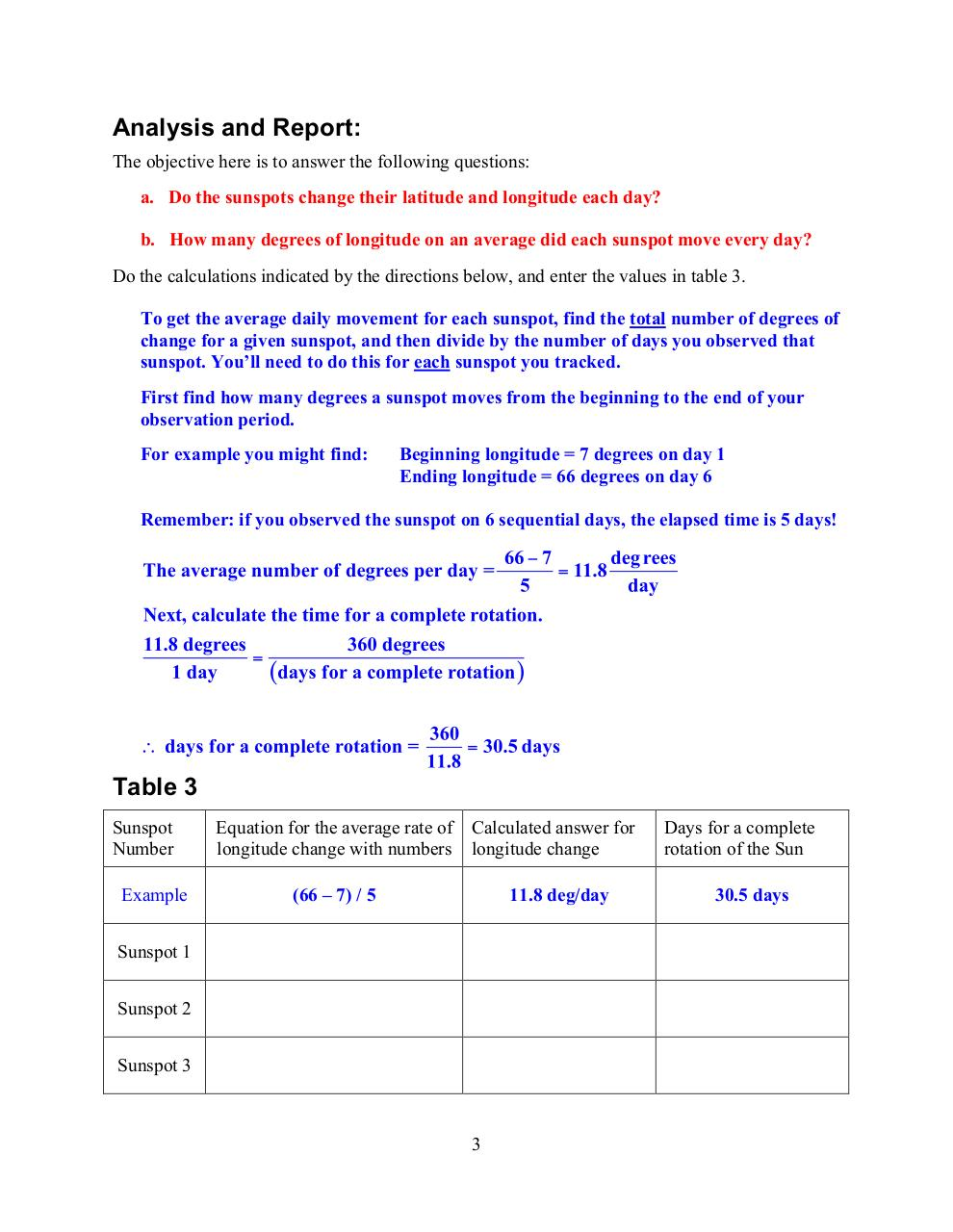Sunspots and the Rotation of the Sun.pdfPage 1 2 34521

Text preview

Analysis and Report:
The objective here is to answer the following questions:
a. Do the sunspots change their latitude and longitude each day?
b. How many degrees of longitude on an average did each sunspot move every day?
Do the calculations indicated by the directions below, and enter the values in table 3.
To get the average daily movement for each sunspot, find the total number of degrees of
change for a given sunspot, and then divide by the number of days you observed that
sunspot. You’ll need to do this for each sunspot you tracked.
First find how many degrees a sunspot moves from the beginning to the end of your
observation period.
For example you might find:

Beginning longitude = 7 degrees on day 1
Ending longitude = 66 degrees on day 6

Remember: if you observed the sunspot on 6 sequential days, the elapsed time is 5 days!

Table 3
Sunspot
Number
Example

Equation for the average rate of Calculated answer for
longitude change with numbers longitude change
(66 – 7) / 5

11.8 deg/day

Sunspot 1
Sunspot 2
Sunspot 3

3

Days for a complete
rotation of the Sun
30.5 days